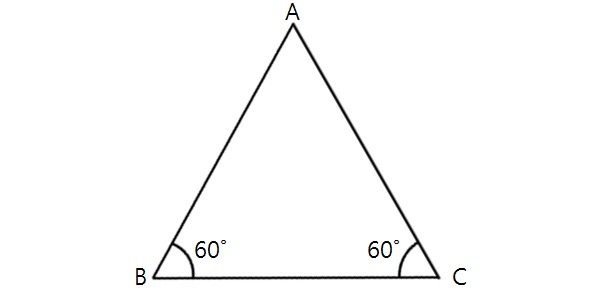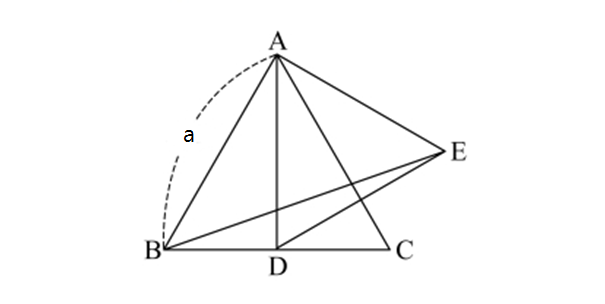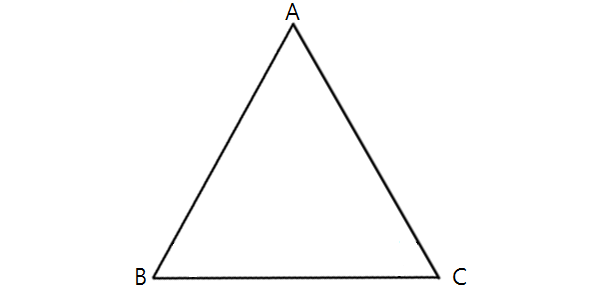Geometry

# Properties of Equilateral TrianglesIn the above diagram, $\angle ABC=60^\circ$ and $\angle ACB=60^\circ.$ If $\lvert \overline{AB}\rvert =3,$ what is the area of $\triangle ABC$?

Given two distinct points $A$ and $B$ in the plane, how many distinct points $C$ are there on the same plane such that $\triangle ABC$ is an equilateral triangle?

There are $8$ equilateral triangles each of which is $4 \text{ cm}$ on a side. All of these triangles have been made by bending copper wires. Now, you unbend the wires and try to make squares with side length $1 \text{ cm}.$ How many such squares can you make?In the above diagram, the side length of equilateral triangle $\triangle ABC$ is $a=3.$ If $D$ is the midpoint of $\overline{BC}$ and $\triangle ADE$ is also an equilateral triangle, what is the area of $\triangle ABE$?In the above diagram, the triangle is the equilateral triangle. If $\overline{AB}=5.$ What is the height of $\triangle ABC$?

×